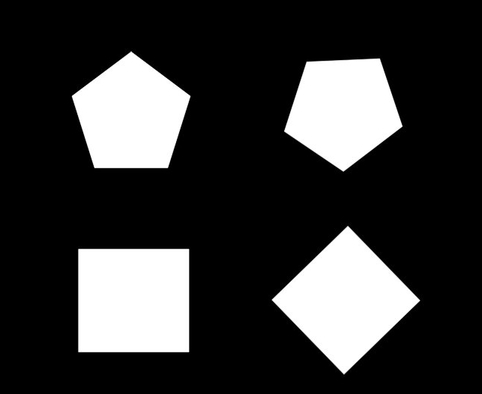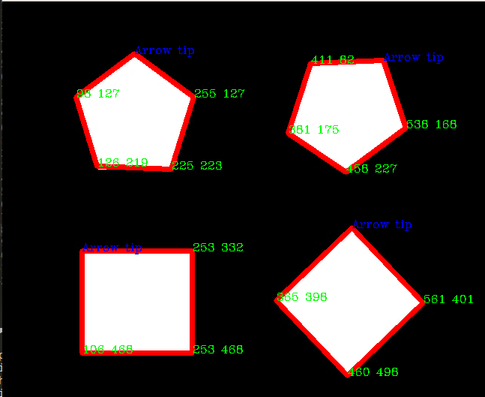# Find Co-ordinates of Contours using OpenCV | Python

• Difficulty Level : Hard
• Last Updated : 13 Oct, 2019

In this article, we will learn how to find the co-ordinates of Contours with the help of OpenCV. Contours are defined as the line joining all the points along the boundary of an image that are having the same intensity. Contours come handy in shape analysis, finding the size of the object of interest, and object detection.

We will be using OpenCV `findContour()` function that helps in extracting the contours from the image.

Approach :
The Co-ordinates of each vertices of a contour is hidden in the contour itself. In this approach, we will be using numpy library to convert all the co-ordinates of a contour into a linear array. This linear array would contain the x and y co-ordinates of each contour. The key point here is that the first co-ordinate in the array would always be the co-ordinate of the topmost vertex and hence could help in detection of orientation of an image.

In the following code, we will be using an image named ‘test.jpg’ to find contours and print the co-ordinates of the vertices on the image itself.

 `# Python code to find the co-ordinates of``# the contours detected in an image.``import` `numpy as np``import` `cv2`` ` `# Reading image``font ``=` `cv2.FONT_HERSHEY_COMPLEX``img2 ``=` `cv2.imread(``'test.jpg'``, cv2.IMREAD_COLOR)`` ` `# Reading same image in another ``# variable and converting to gray scale.``img ``=` `cv2.imread(``'test.jpg'``, cv2.IMREAD_GRAYSCALE)`` ` `# Converting image to a binary image``# ( black and white only image).``_, threshold ``=` `cv2.threshold(img, ``110``, ``255``, cv2.THRESH_BINARY)`` ` `# Detecting contours in image.``contours, _``=` `cv2.findContours(threshold, cv2.RETR_TREE,``                               ``cv2.CHAIN_APPROX_SIMPLE)`` ` `# Going through every contours found in the image.``for` `cnt ``in` `contours :`` ` `    ``approx ``=` `cv2.approxPolyDP(cnt, ``0.009` `*` `cv2.arcLength(cnt, ``True``), ``True``)`` ` `    ``# draws boundary of contours.``    ``cv2.drawContours(img2, [approx], ``0``, (``0``, ``0``, ``255``), ``5``) `` ` `    ``# Used to flatted the array containing``    ``# the co-ordinates of the vertices.``    ``n ``=` `approx.ravel() ``    ``i ``=` `0`` ` `    ``for` `j ``in` `n :``        ``if``(i ``%` `2` `=``=` `0``):``            ``x ``=` `n[i]``            ``y ``=` `n[i ``+` `1``]`` ` `            ``# String containing the co-ordinates.``            ``string ``=` `str``(x) ``+` `" "` `+` `str``(y) `` ` `            ``if``(i ``=``=` `0``):``                ``# text on topmost co-ordinate.``                ``cv2.putText(img2, ``"Arrow tip"``, (x, y),``                                ``font, ``0.5``, (``255``, ``0``, ``0``)) ``            ``else``:``                ``# text on remaining co-ordinates.``                ``cv2.putText(img2, string, (x, y), ``                          ``font, ``0.5``, (``0``, ``255``, ``0``)) ``        ``i ``=` `i ``+` `1`` ` `# Showing the final image.``cv2.imshow(``'image2'``, img2) `` ` `# Exiting the window if 'q' is pressed on the keyboard.``if` `cv2.waitKey(``0``) & ``0xFF` `=``=` `ord``(``'q'``): ``    ``cv2.destroyAllWindows()`

Input image:Output:My Personal Notes arrow_drop_up